# 真实世界网络结构 (Structure of Real-World Network)

## 分支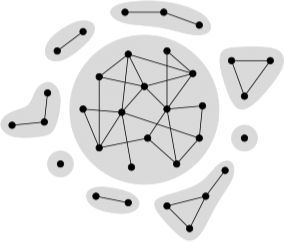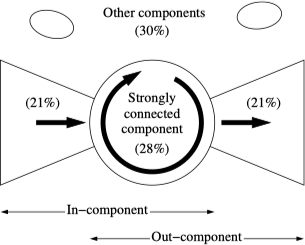## 度分布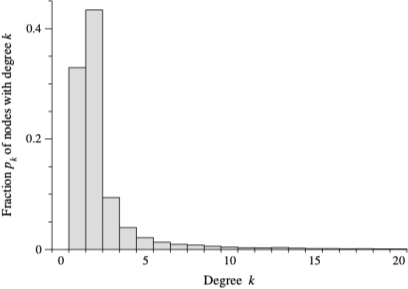## 幂律和无标度网络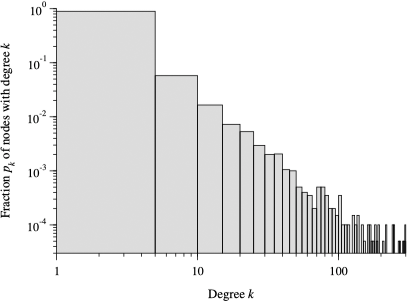$$\ln p_k = - \alpha \ln k + c$$

$$p_k = C k^{- \alpha}$$

$$P_k = \sum_{k' = k}^{\infty} p_{k'}$$

$$P_{k}=C \sum_{k^{\prime}=k}^{\infty} k^{\prime-\alpha} \simeq C \int_{k}^{\infty} k^{\prime-\alpha} \mathrm{d} k^{\prime}=\frac{C}{\alpha-1} k^{-(\alpha-1)}$$

## 聚类系数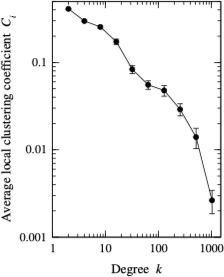1. Newman, M. E. J. (2014) 网络科学引论. 电子工业出版社. ↩︎